# Principal component analysis

14 de Mar de 2015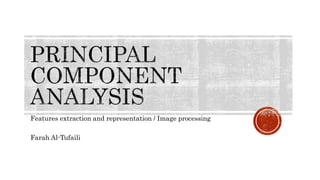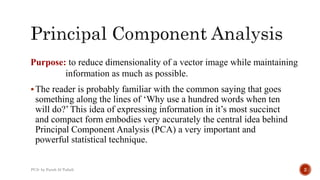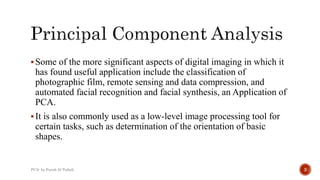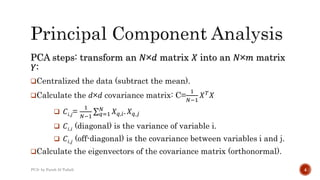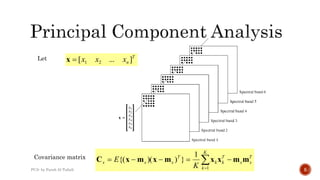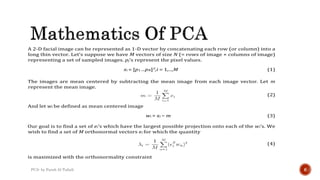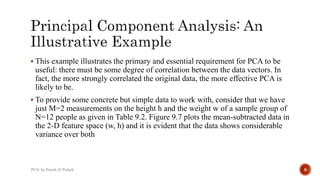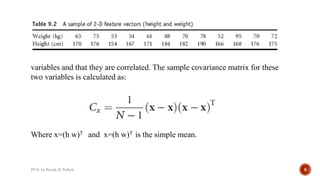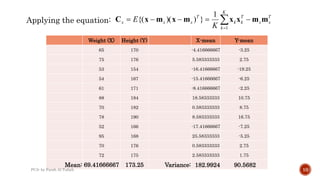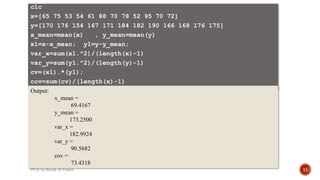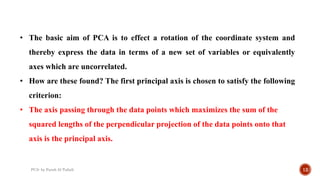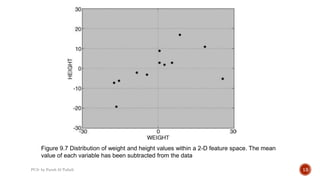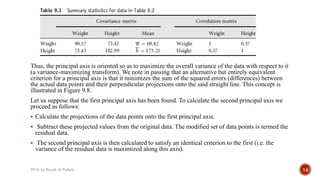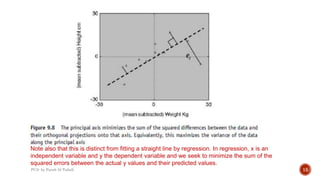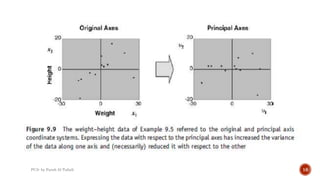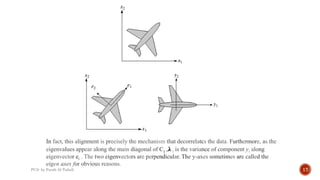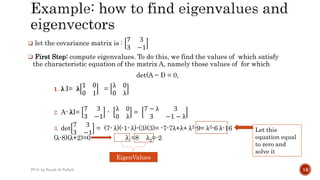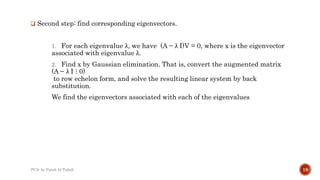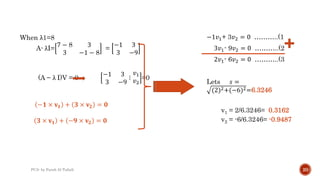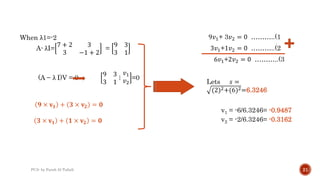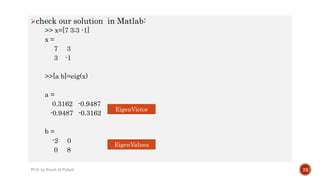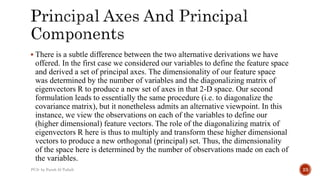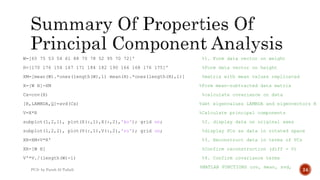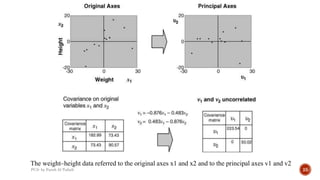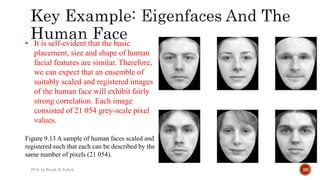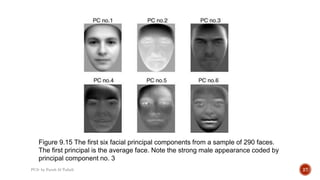1 de 27

### Principal component analysis

• 1. Features extraction and representation / Image processing Farah Al-Tufaili
• 2. The reader is probably familiar with the common saying that goes something along the lines of ‘Why use a hundred words when ten will do?’ This idea of expressing information in it’s most succinct and compact form embodies very accurately the central idea behind Principal Component Analysis (PCA) a very important and powerful statistical technique. Purpose: to reduce dimensionality of a vector image while maintaining information as much as possible. PCA- by Farah Al-Tufaili 2
• 3. Some of the more significant aspects of digital imaging in which it has found useful application include the classification of photographic film, remote sensing and data compression, and automated facial recognition and facial synthesis, an Application of PCA. It is also commonly used as a low-level image processing tool for certain tasks, such as determination of the orientation of basic shapes. PCA- by Farah Al-Tufaili 3
• 4. PCA steps: transform an 𝑁×𝑑 matrix 𝑋 into an 𝑁×𝑚 matrix 𝑌: Centralized the data (subtract the mean). Calculate the 𝑑×𝑑 covariance matrix: C= 1 𝑁−1 𝑋 𝑇 𝑋  𝐶𝑖,𝑗= 1 𝑁−1 𝑞=1 𝑁 𝑋 𝑞,𝑖. 𝑋 𝑞,𝑗  𝐶𝑖,𝑖 (diagonal) is the variance of variable i.  𝐶𝑖,𝑗 (off-diagonal) is the covariance between variables i and j. Calculate the eigenvectors of the covariance matrix (orthonormal). PCA- by Farah Al-Tufaili 4
• 5. Let Covariance matrix   K k T xx T kk T xxx K E 1 1 }))({( mmxxmxmxC T nxxx ]...[ 21x PCA- by Farah Al-Tufaili 5
• 6. A 2-D facial image can be represented as 1-D vector by concatenating each row (or column) into a long thin vector. Let’s suppose we have M vectors of size N (= rows of image × columns of image) representing a set of sampled images. pj’s represent the pixel values. xi = [p1 ...pN]T,i = 1,...,M (1) The images are mean centered by subtracting the mean image from each image vector. Let m represent the mean image. (2) And let wi be defined as mean centered image wi = xi − m (3) Our goal is to find a set of ei’s which have the largest possible projection onto each of the wi’s. We wish to find a set of M orthonormal vectors ei for which the quantity (4) is maximized with the orthonormality constraint PCA- by Farah Al-Tufaili 6
• 7.  This example illustrates the primary and essential requirement for PCA to be useful: there must be some degree of correlation between the data vectors. In fact, the more strongly correlated the original data, the more effective PCA is likely to be.  To provide some concrete but simple data to work with, consider that we have just M=2 measurements on the height h and the weight w of a sample group of N=12 people as given in Table 9.2. Figure 9.7 plots the mean-subtracted data in the 2-D feature space (w, h) and it is evident that the data shows considerable variance over both PCA- by Farah Al-Tufaili 8
• 8. variables and that they are correlated. The sample covariance matrix for these two variables is calculated as: Where x=(h w)T and x=(h w)T is the simple mean. PCA- by Farah Al-Tufaili 9
• 9. Weight (X) Height (Y) X-mean Y-mean 65 170 -4.416666667 -3.25 75 176 5.583333333 2.75 53 154 -16.41666667 -19.25 54 167 -15.41666667 -6.25 61 171 -8.416666667 -2.25 88 184 18.58333333 10.75 70 182 0.583333333 8.75 78 190 8.583333333 16.75 52 166 -17.41666667 -7.25 95 168 25.58333333 -5.25 70 176 0.583333333 2.75 72 175 2.583333333 1.75 Mean: 69.41666667 173.25 Variance: 182.9924 90.5682 Applying the equation:   K k T xx T kk T xxx K E 1 1 }))({( mmxxmxmxC PCA- by Farah Al-Tufaili 10
• 10. clc x=[65 75 53 54 61 88 70 78 52 95 70 72] y=[170 176 154 167 171 184 182 190 166 168 176 175] x_mean=mean(x) , y_mean=mean(y) x1=x-x_mean; y1=y-y_mean; var_x=sum(x1.^2)/(length(x)-1) var_y=sum(y1.^2)/(length(y)-1) cv=(x1).*(y1); cov=sum(cv)/(length(x)-1) Output: x_mean = 69.4167 y_mean = 173.2500 var_x = 182.9924 var_y = 90.5682 cov = 73.4318 PCA- by Farah Al-Tufaili 11
• 11. • The basic aim of PCA is to effect a rotation of the coordinate system and thereby express the data in terms of a new set of variables or equivalently axes which are uncorrelated. • How are these found? The first principal axis is chosen to satisfy the following criterion: • The axis passing through the data points which maximizes the sum of the squared lengths of the perpendicular projection of the data points onto that axis is the principal axis. PCA- by Farah Al-Tufaili 12
• 12. Figure 9.7 Distribution of weight and height values within a 2-D feature space. The mean value of each variable has been subtracted from the data PCA- by Farah Al-Tufaili 13
• 13. Thus, the principal axis is oriented so as to maximize the overall variance of the data with respect to it (a variance-maximizing transform). We note in passing that an alternative but entirely equivalent criterion for a principal axis is that it minimizes the sum of the squared errors (differences) between the actual data points and their perpendicular projections onto the said straight line. This concept is illustrated in Figure 9.8. Let us suppose that the first principal axis has been found. To calculate the second principal axis we proceed as follows:  Calculate the projections of the data points onto the first principal axis.  Subtract these projected values from the original data. The modified set of data points is termed the residual data.  The second principal axis is then calculated to satisfy an identical criterion to the first (i.e. the variance of the residual data is maximized along this axis). PCA- by Farah Al-Tufaili 14
• 14. Note also that this is distinct from fitting a straight line by regression. In regression, x is an independent variable and y the dependent variable and we seek to minimize the sum of the squared errors between the actual y values and their predicted values. PCA- by Farah Al-Tufaili 15
• 15. PCA- by Farah Al-Tufaili 16
• 16. In fact, this alignment is precisely the mechanism that decorrelatcs the data. Furthermore, as the eigenvalues appear along the main diagonal of Cy ,λ i is the variance of component yi along eigenvector ei . The two eigenvectors are perpendicular. The y-axes sometimes are called the eigen axes for obvious reasons. PCA- by Farah Al-Tufaili 17
• 17.  let the covariance matrix is : 7 3 3 −1  First Step: compute eigenvalues. To do this, we find the values of which satisfy the characteristic equation of the matrix A, namely those values of for which det(A − I) = 0, 1. λ I= λ 1 0 0 1 = λ 0 0 λ 2. A- λI= 7 3 3 −1 - λ 0 0 λ = 7 − λ 3 3 −1 − λ 3. det 7 3 3 −1 = (7- λ)(-1- λ)-(3)(3)= -7-7λ+λ+ λ2-9= λ2-6 λ-16 (λ-8)(λ+2)=0 λ1=8 λ2=-2 EigenValues Let this equation equal to zero and solve it PCA- by Farah Al-Tufaili 18
• 18.  Second step: find corresponding eigenvectors. 1. For each eigenvalue λ, we have (A − λ I)V = 0, where x is the eigenvector associated with eigenvalue λ. 2. Find x by Gaussian elimination. That is, convert the augmented matrix (A − λ I ⋮ 0) to row echelon form, and solve the resulting linear system by back substitution. We find the eigenvectors associated with each of the eigenvalues PCA- by Farah Al-Tufaili 19
• 19. −1𝑣1+ 3𝑣2 = 0 ………..(1 3𝑣1- 9𝑣2 = 0 ………..(2 2𝑣1- 6𝑣2 = 0 ………..(3 Lets 𝑠 = (2)2+(−6)2=6.3246 v1 = 2/6.3246= 0.3162 v2 = -6/6.3246= -0.9487 PCA- by Farah Al-Tufaili 20 When λ1=8 A- λI= 7 − 8 3 3 −1 − 8 = −1 3 3 −9 (A − λ I)V = 0 −1 3 3 −9 ⋮ 𝑣1 𝑣2 =0 −𝟏 × 𝐯 𝟏 + 𝟑 × 𝐯 𝟐 = 𝟎 𝟑 × 𝐯 𝟏 + −𝟗 × 𝐯 𝟐 = 𝟎
• 20. PCA- by Farah Al-Tufaili 21 9𝑣1+ 3𝑣2 = 0 ………..(1 3𝑣1+1𝑣2 = 0 ………..(2 6𝑣1+2𝑣2 = 0 ………..(3 Lets 𝑠 = (2)2+(6)2=6.3246 v1 = -6/6.3246= -0.9487 v2 = -2/6.3246= -0.3162 When λ1=-2 A- λI= 7 + 2 3 3 −1 + 2 = 9 3 3 1 (A − λ I)V = 0 9 3 3 1 ⋮ 𝑣1 𝑣2 =0 𝟗 × 𝐯 𝟏 + 𝟑 × 𝐯 𝟐 = 𝟎 𝟑 × 𝐯 𝟏 + 𝟏 × 𝐯 𝟐 = 𝟎
• 21. check our solution in Matlab: >> x=[7 3;3 -1] x = 7 3 3 -1 >>[a b]=eig(x) a = 0.3162 -0.9487 -0.9487 -0.3162 b = -2 0 0 8 PCA- by Farah Al-Tufaili 22 EigenValues EigenVictor
• 22.  There is a subtle difference between the two alternative derivations we have offered. In the first case we considered our variables to define the feature space and derived a set of principal axes. The dimensionality of our feature space was determined by the number of variables and the diagonalizing matrix of eigenvectors R to produce a new set of axes in that 2-D space. Our second formulation leads to essentially the same procedure (i.e. to diagonalize the covariance matrix), but it nonetheless admits an alternative viewpoint. In this instance, we view the observations on each of the variables to define our (higher dimensional) feature vectors. The role of the diagonalizing matrix of eigenvectors R here is thus to multiply and transform these higher dimensional vectors to produce a new orthogonal (principal) set. Thus, the dimensionality of the space here is determined by the number of observations made on each of the variables. PCA- by Farah Al-Tufaili 23
• 23. W=[65 75 53 54 61 88 70 78 52 95 70 72]' %1. Form data vector on weight H=[170 176 154 167 171 184 182 190 166 168 176 175]' %Form data vector on height XM=[mean(W).*ones(length(W),1) mean(H).*ones(length(H),1)] %matrix with mean values replicated X=[W H]-XM %Form mean-subtracted data matrix Cx=cov(X) %calculate covariance on data [R,LAMBDA,Q]=svd(Cx) %Get eigenvalues LAMBDA and eigenvectors R V=X*R %Calculate principal components subplot(1,2,1), plot(X(:,1),X(:,2),'ko'); grid on; %2. display data on original axes subplot(1,2,2), plot(V(:,1),V(:,2),'ro'); grid on; %display PCs as data in rotated space XR=XM+V*R' %3. Reconstruct data in terms of PCs XR-[W H] %Confirm reconstruction (diff = 0) V'*V./(length(W)-1) %4. Confirm covariance terms %MATLAB FUNCTIONS cov, mean, svd, PCA- by Farah Al-Tufaili 24
• 24. The weight–height data referred to the original axes x1 and x2 and to the principal axes v1 and v2 PCA- by Farah Al-Tufaili 25
• 25. • It is self-evident that the basic placement, size and shape of human facial features are similar. Therefore, we can expect that an ensemble of suitably scaled and registered images of the human face will exhibit fairly strong correlation. Each image consisted of 21 054 grey-scale pixel values. Figure 9.13 A sample of human faces scaled and registered such that each can be described by the same number of pixels (21 054). PCA- by Farah Al-Tufaili 26
• 26. Figure 9.15 The first six facial principal components from a sample of 290 faces. The first principal is the average face. Note the strong male appearance coded by principal component no. 3 PCA- by Farah Al-Tufaili 27
• 27. Any Questions? PCA- by Farah Al-Tufaili 28